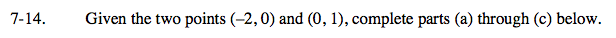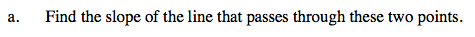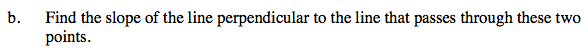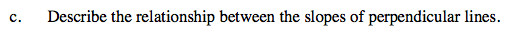### Home > A2C > Chapter 7 > Lesson 7.1.1 > Problem7-14

7-14.$\text{Remember that the slope } = \frac{\Delta y}{\Delta x}.$

$m = \frac{1}{2}$The slopes of perpendicular lines are opposite reciprocals of each other.

$\text{Example: lines with slopes of } - \frac{2}{3} \text{ and } \frac{3}{2}.$

Remember: the product of opposite reciprocals is −1.

The slope is −2.See part (b).